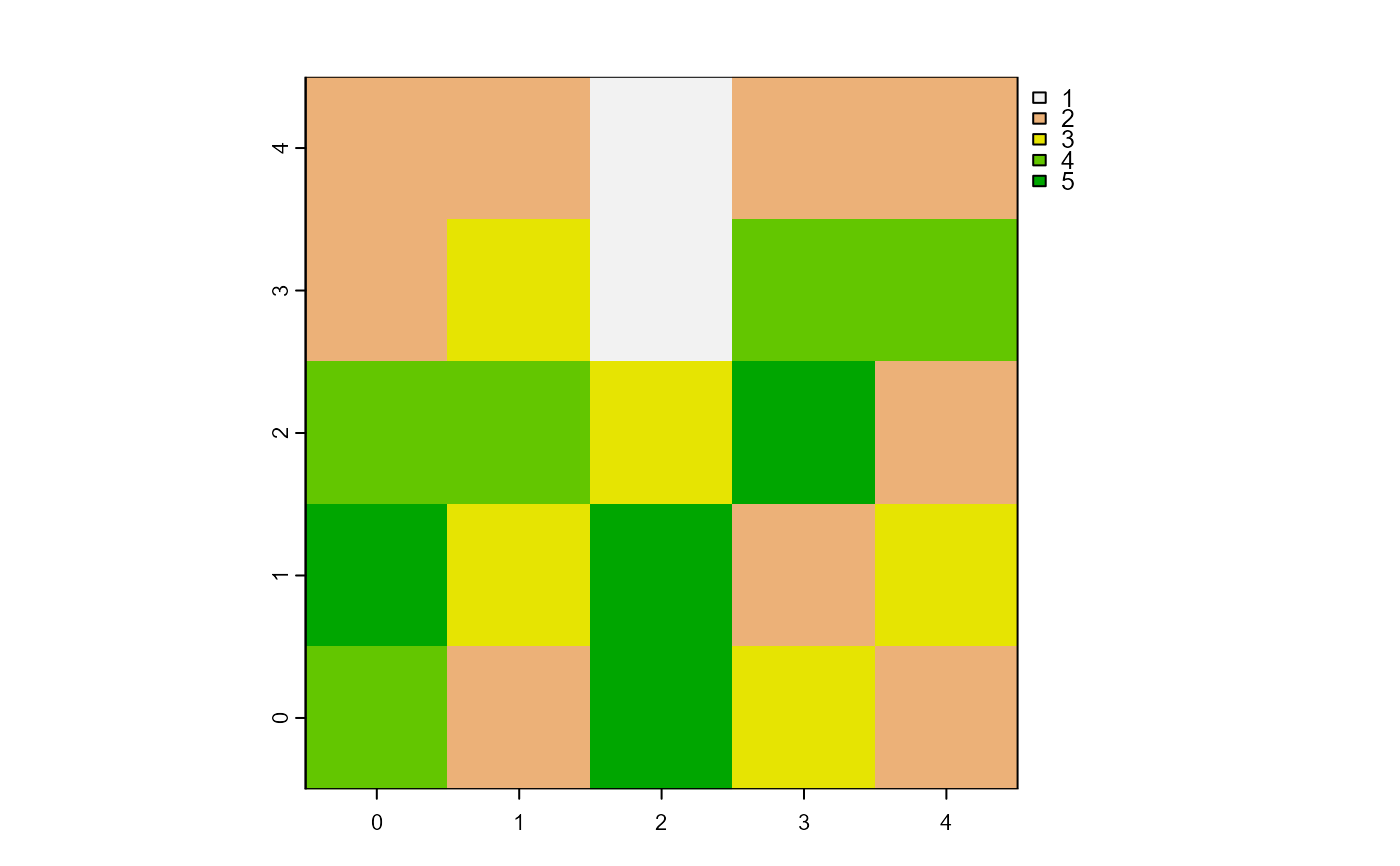Report the patches or turtles among agents which have their variable equals to the maximum value.

withMax(agents, world, var)

# S4 method for matrix,worldMatrix,missing
withMax(agents, world)

# S4 method for matrix,worldArray,character
withMax(agents, world, var)

# S4 method for agentMatrix,missing,character
withMax(agents, var)

## Arguments

agents

Matrix (ncol = 2) with the first column pxcor and the second column pycor representing the patches coordinates, or

AgentMatrix object representing the moving agents.

world

WorldMatrix or worldArray object.

var

Character. The name of the selected agents variable. If agents are patches and the world is a worldMatrix object, var must not be provided. If agents are patches and the world is a worldArray object, var is the name of the layer to use to define the patches values. If agents are turtles, var is one of the turtles' variable and can be equal to xcor, ycor, any of the variables created when turtles were created, as well as any variable created using turtlesOwn().

## Value

Matrix (ncol = 2) with the first column pxcor and the second column pycor representing the coordinates of the patches among the agents which have their variable equal to the maximum value among the agents, or AgentMatrix representing the turtles among the agents which have their variable var equal to the maximum value among the agents.

## Details

world must not be provided if agents are turtles.

Sarah Bauduin

## Examples

# Patches
w1 <- createWorld(minPxcor = 0, maxPxcor = 4, minPycor = 0, maxPycor = 4,
data = sample(1:5, size = 25, replace = TRUE))
plot(w1)p1 <- withMax(agents = patches(w1), world = w1)

# Turtles
t1 <- createTurtles(n = 10, coords = randomXYcor(w1, n = 10),
heading = sample(1:3, size = 10, replace= TRUE))
t2 <- withMax(agents = t1, var = "heading")